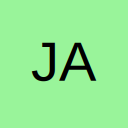My function needs to know what cell, row and column it is in?

• Hi,

I am looking for some VBA that will tell my function what cell it is in, for example:

Code
1. function wibble()
2. wibble = ("I am in cell " & X & "or column " & 1 & " and row " & 2)
3. End function

The output would be: 1 am in cell A1 or column 1 and row 1

On an Excel worksheet the equivalent is "=ROW()" or "=COLUMN()" but there does not appear to be an application.worksheetfunction equivalent.

Many thanks

James

• Re: My function needs to know what cell, row and column it is in?

Can only assume you want the address of the active cell

Code
1. MsgBox "I am in cell " & ActiveCell.Address
2. MsgBox "I am in Column " & ActiveCell.Column & " and row " & ActiveCell.Row
• Re: My function needs to know what cell, row and column it is in?

Code
1. Function wibble()
2. If TypeOf Application.Caller Is Range Then
3. wibble = "I am in cell " & Application.Caller.Address(0, 0)
4. End If
5. End Function

?

• Re: My function needs to know what cell, row and column it is in?

Many thanks!